# Assignment 5 on heat and thermodynamics (objective questions)

Multiple choice questions with one or more answers
Q- 1: It is known that curves A, B, C are Isobaric, Isothermal, Adiabatic process then when one is correct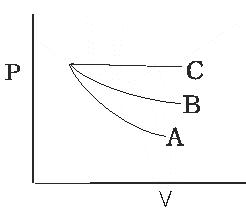a, A - Adiabatic, B - Isothermal,, C - Isobaric
b, A- Isothermal,, B - Adiabatic, C - Isobaric
c, A - Isobaric, B - Isothermal C - Adiabatic
d, None of these

Q- 2: Which of the following graph correctly represent the variation
δ = dV /V dT
for an ideal gas at constant pressure
a.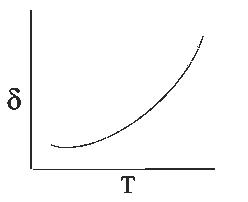b.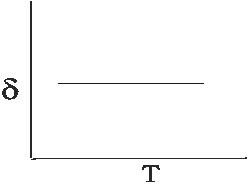c.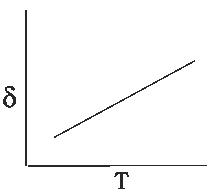d.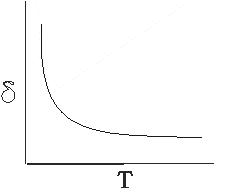Q- 3: An ideal gas taken round the cycle ABCA as shown in PV diagram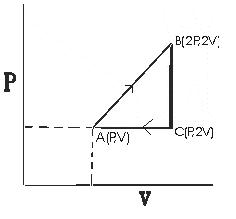The work done during the cycle,
a. PV
b. PV/2
c. 2PV
d. PV/3

Q- 4. Consider the following statements

(Assertion) The internal energy of an ideal gas does not change during an Isothermal process
(Reason) The decrease in the volume of the gas is compensated by a corresponding increase in pressure when its temperature is constant in accordance with Boyle law

a, Both A & R are true and R is correct Explanation of A
b, Both A & R are true and R is not correct Explanation of A
c, A is true R is false
d, A is false but R is true

Q- 5:
A thermally insulated vessel containing an gas when molar mass is M and Ratio of specific heat Cp / Cv = γ move with a velocity V.The gas temperature increase by ΔT due to sudden stoppage of vessel. Find the value of V in terms of ΔT, M, γ
a, V = [2RΔT / M (γ-1)]1/2
b, V = [2RΔT / M (γ+1)]1/2
c, V = [2RΔT / M (γ-1)1/2]1/2
d, V = [2RΔT / M (γ+1)1/2]1/2

Q- 6:Match the column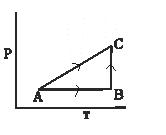1, Isobaric process a, AC
2, Isochoric Process b, AB
3, Isothermal Process c, BC

Q-7:
An ideal gas is taken through a cyclic thermodynamics process through four steps.
The amount of heat involved in the steps are Q1 = 5960 J,Q2 = - 5600 J,Q3= -3000 J,Q4 = -3600 J
respectively. The corresponding quantities of Internal energy changes are ΔU1 = 3.760 J ,ΔU2 = - 4800 J,ΔU3 = -1800 J,ΔU4 = ?
find the value ΔU4 & net work done
a, 2930 J, 960 J
b, 2830 J, 900 J
C, 2930 J, -960 J
d, -2930 J, 960 J

Q-8:
An ideal gas who ratio of specific heat Cp / Cv = γ is Expanded according to the law P = av2
when a is constant. The ratio of final volume to Initial value is n .find the ΔU.Intial volume is V0
a, aV03 (n3-1) / γ-1
b. aV02 (n2 - 1) / γ-1
c, aV03 (n3 + 1) /γ+1
d, aV02 (n2 + 1) /γ-1

Q-9: During an adiabatic process the square of the pressure of a gas is proportional to the fifth power of its absolute temperature. The ratio of specific heat Cp / Cv for that gas is
a. 3/5
b. 4/3
c. 5/3
d. 3/2

Q-10: A vessel contains 4 mole of O2(relative molar mass 32) at temperature T. The pressure is P. An identical vessel contains 1 mole of nitrogen of temperature 2T. find the pressure the
a P/2
b 2P
c 8P
d P

Q-11:
What is the molar specific heat of a isothermal & adiabatic process respectively
a, ∞ , 0
b, 0, ∞
c. 0, 0
d, none of these

Q-12: An ideal gas has molar specific heat at constant volume = Cv. find the molar heat capacity of this gas as a function of volume V at the gas goes through process.
T = T0(eaV + 1)
a, Cv + (R/aV) + (R/aVeaV)
b, Cv + (R/aV)
c, Cv+ (R2/aV)
d, Cv - (R2/aV)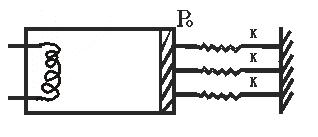1 mole of a diatomic idea gas is enclosed in a adiabatic cylinder filled with a smooth light adiabatic Piston. The Piston is connected to three spring of spring constant K as shown in figure. The area of crossection of Cylinder is A Initially spring is in its natural length and atmosphere pressure is P0

Q-13: find the pressure of the gas
a, P0
b, 2P0
c, P0/2
d, 4P0

Q-14: If the heat is supplied to the gas and piston move by distance L due to that then what is the work done by the gas
a, P0AL+ (3/2)KL2
b, P0AL
c, (3/2)KL2
d, P0AL - (3/2)KL2

Q-15: if Temp changes by ΔT due to heat transfer and γ=5/2 then Find out the internal energy change
a, RΔT
b, (2/3)RΔT
c, (5/2)RΔT
d, RΔT/2

Q-16: Find the total heat supply
a, RΔT +(3/2)KL2
b, P0AL+(3/2)KL2+ (2/3)RΔT
c, RΔT -(3/2)KL2
d, RΔT + P0AL

Multiple choice questions with one or more answers
Q-17: One mole of ideal gas having adiabatic coefficient γ1 is mixed with 1 one mole of an ideal gas have adiabatic coefficient γ2
Find the γ of the mixture
a,[(2γ1γ21 - γ2 ) /(γ1 + γ2 - 2)]
b.[(2γ1γ21 - γ2 ) /(γ1 + γ2 + 2)]
c.[(2γ1γ212 ) /(γ1 + γ2 - 2)]
d. None of these

Q-18:
We have a process defined as PVn = constant
and we have an adiabatic process defined by PVγ = Constant
and so thermal process defined as PV = Constant
find the Ratio of Bulk modules of Polytropic, adiabatic, isothermal process
a, n:γ:1
b, 1:n:γ
c, n22:1
d, 1:n22

Q-19: Find the wok done in the cyclic process as shown in figure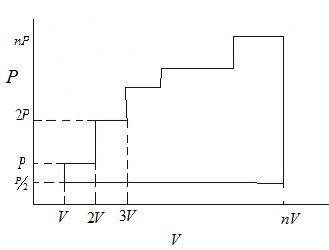a. n2PV/2
b. n2PV/8
c. n2PV/16
d. none of these

Q-20: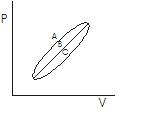Let Q1, Q2 , Q2 heat given to the system in processes A, B, C respectively
consider two statements

a, Q1 > Q2 > Q3
b, WA > WB > WC

1, Both A & B are Correct
2, Both A & B are wrong
3. A is Correct Only
4. B is Correct Only.

Q-21:
Match the Column
a, Isothermal process x ΔU = ΔQ
b, Adiabatic Process Y ΔU = ΔQ - ΔW
c, Isobaric process Z ΔU = -ΔW
d, Isochoric process W ΔQ = ΔW

a, a -> x, b-> y, c->z, d->w
b, a->w, b->z, c->x, d->y
c, a->y, b->x, c->z, d->w
d, a->z,b->w,c->y,d->x

Solutions

Note to our visitors :-

Thanks for visiting our website. From feedback of our visitors we came to know that sometimes you are not able to see the answers given under "Answers" tab below questions. This might happen sometimes as we use javascript there. So you can view answers where they are available by reloding the page and letting it reload properly by waiting few more seconds before clicking the button.
We really do hope that this resolve the issue. If you still hare facing problems then feel free to contact us using feedback button or contact us directly by sending is an email at [email protected]
We are aware that our users want answers to all the questions in the website. Since ours is more or less a one man army we are working towards providing answers to questions available at our website.